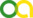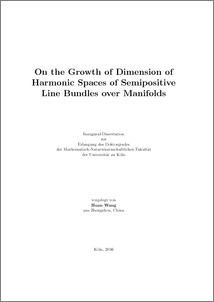Wang, Huan (2016). On the Growth of Dimension of Harmonic Spaces of Semipositive Line Bundles over Manifolds. PhD thesis, Universität zu Köln.All available versions of this item

• On the Growth of Dimension of Harmonic Spaces of Semipositive Line Bundles over Manifolds. (deposited 28 Jul 2016 14:05) [Currently Displayed]Preview
PDF
Thesis_HuanWang_Library.pdf

Abstract

This thesis consists of six parts. In the first part, we give a general introduction of our topics. In the second part, we study the harmonic space of line bundle valued forms over a covering manifold with a discrete group action, and obtain an asymptotic estimate for the von Neumann dimension of the space of harmonic \$(n,q)\$-forms with values in high tensor powers of a semipositive line bundle. In particular, we estimate the von Neumann dimension of the corresponding reduced \$L^2\$-Dolbeault cohomology group. The main tool is a local estimate of the pointwise norm of harmonic forms with values in semipositive line bundles over Hermitian manifolds. In the third part, we study the holomorphic extension problem of smooth forms with values in holomorphic vector bundles from the boundary of a pseudo-concave domain, which is in a compact Hermitian manifold associated with a holomorphic line bundle. We prove the existence of the meromorphic extension of \$\ddbar_b\$-closed \$(n,q+1)\$-forms with values in holomorphic vector bundles, when the domain is \$q\$-concave and the line bundle is semi-positive everywhere and positive at one point. In the fourth part, we study the \$L^2\$ holomorphic functions on hyperconcave ends and prove that the dimension of the space of \$L^2\$ holomorphic functions on hyperconcave ends is infinite. The main tool is the construction of \$L^2\$-peak functions at boundary points by using the solution of \$\ddbar\$-Neumann problem of Kohn and the compactification theorem of Marinescu-Dinh. In the fifth part, we give a remark on the Bergman kernel of symmetric tensor power of trivial vector bundles on compact Hermitian manifold by the Theorem of Le Potier. In the last part, we study the \$\ddbar\$-equation on \$\C^n\$ with growing weights, and generalize a related result of Hedenmalm on \$\C\$.

Item Type: Thesis (PhD thesis)
Translated abstract:
AbstractLanguage
Die Doktorarbeit ist in sechs Teile unterteilt. Im ersten Teil wird eine Einführung in die behandelten Themen gegeben. Im zweiten Teil wird der Raum der harmonischen Formen auf einem Linienbündel über einer Überdeckungsmannigfaltigkeit mit einer diskreten Gruppenwirkung untersucht und eine asymptotische Abschätzung für die von Neumann-Dimension des Raumes der harmonischen \$(n,q)\$-Formen mit Werten in den hohen Tensorprodukten eines semipositiven Linienbündels bewiesen. Insbesondere wird die von Neumann Dimension der entsprechenden reduzierten \$L^2\$-Dolbeault Kohomologiegruppe abgeschätzt. Das wichtigste Werkzeug dabei ist eine lokale Abschätzung der punktweisen Norm von harmonischen Formen mit Werten in semipositiven Linienbündeln über hermitischen Mannigfaltigkeiten. Im dritten Teil wird das Problem der holomorphen Erweiterung von glatten Formen mit Werten in holomorphen Vektorbündeln vom Rand eines pseudo-konkaven Gebiets behandelt, das in einer kompakten hermitische Mannigfaltigkeit liegt, die mit einem holomorphen Linienbündel assoziiert ist. Es wird die Existenz einer meromorphen Erweiterung von \$\ddbar_b\$-geschlossenen \$(n,q+1)\$-Formen mit Werten in holomorphen Vektorbündeln bewiesen unter der Annahme, dass das Gebiet \$q\$-konkav und das Linienbündel überall semipositiv und positiv an einem Punkt ist. Im vierten Teil werden die \$L^2\$ holomorphen Funktionen auf hyperkonkaven Enden studiert und bewiesen, dass die Dimension des Raumes der \$L^2\$ holomorphen Funktionen auf hyperkonkaven Enden unendlich ist. Der wichtigste Schritt ist die Konstruktion von \$L^2\$ maximierenden Funktionen in Randpunkten, wobei die Lösung des \$\ddbar\$-Neumann Problems von Kohn und der Kompaktifizierungssatz von Marinescu-Dinh angewendet wird. Im fünften Teil, wird der Bergman Kern eines symmetrischen Tensorprodukts von trivialen Vektorbündeln auf kompakten Hermitischen Mannigfaltigkeiten mit der Hilfe des Satzes von Le Potier betrachtet. Im letzten Teil, wird die \$\ddbar\$-Gleichung auf \$\C^n\$ mit wachsenden Gewichten studiert und eine verwandtes Resultat von Hedenmal auf \$\C\$ verallgemeinert.German
Creators:
CreatorsEmailORCIDORCID Put Code
Wang, Huanwang@math.uni-koeln.deUNSPECIFIEDUNSPECIFIED
URN: urn:nbn:de:hbz:38-68551
Date: 8 May 2016
Language: English
Faculty: Faculty of Mathematics and Natural Sciences
Divisions: Faculty of Mathematics and Natural Sciences > Department of Mathematics and Computer Science > Mathematical Institute
Subjects: Natural sciences and mathematics
Mathematics
Uncontrolled Keywords:
KeywordsLanguage
Bergman kernel functionEnglish
von Neumann dimensionEnglish
semipositive line bundleEnglish
covering manifoldEnglish
holomorphic extensionEnglish
peak functionEnglish
Date of oral exam: 28 June 2016
Referee:
Marinescu, GeorgeProf. Dr.
Lytchak, AlexanderProf. Dr.
Funders: China Scholarship Council
Refereed: Yes
URI: http://kups.ub.uni-koeln.de/id/eprint/6857View Item